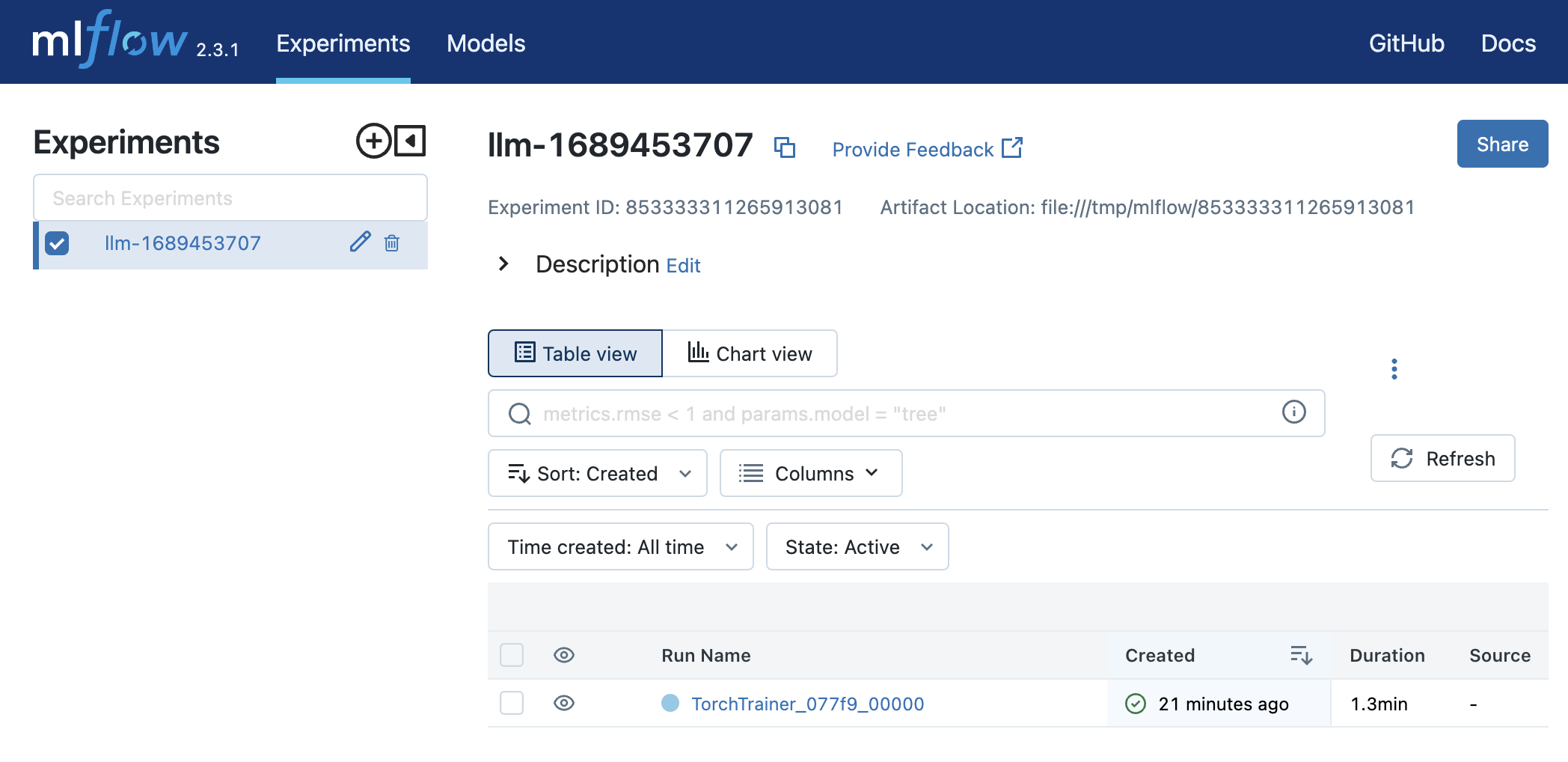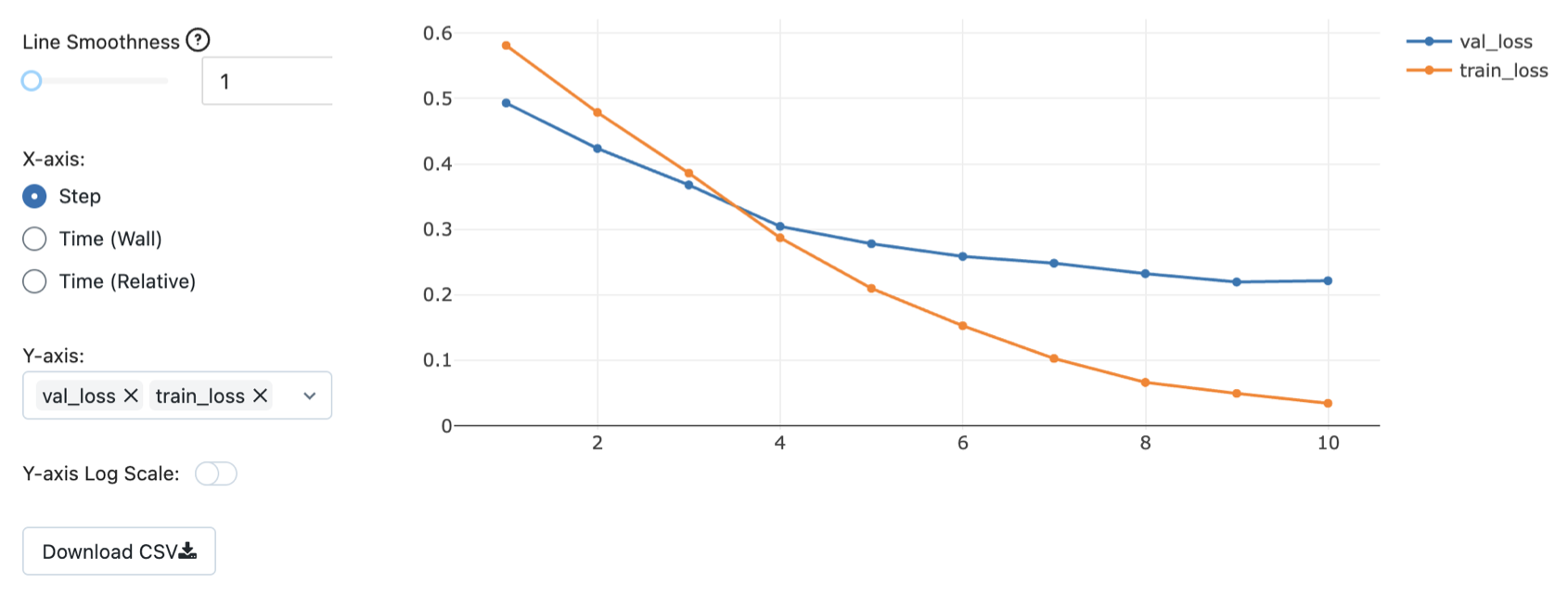# Experiment Tracking

Managing and tracking ML experiments and runs.Goku Mohandas
· ·
Repository · Notebook

📬  Receive new lessons straight to your inbox (once a month) and join 35K+ developers in learning how to responsibly deliver value with ML.

## Intuition

So far, we've been training and evaluating our different baselines but haven't really been tracking these experiments. We'll fix this but defining a proper process for experiment tracking which we'll use for all future experiments (including hyperparameter optimization). Experiment tracking is the processing of managing all the different experiments and their components, such as parameters, metrics, models and other artifacts and it enables us to:

• Organize all the necessary components of a specific experiment. It's important to have everything in one place and know where it is so you can use them later.
• Reproduce past results (easily) using saved experiments.
• Log iterative improvements across time, data, ideas, teams, etc.

## Tools

There are many options for experiment tracking but we're going to use MLFlow (100% free and open-source) because it has all the functionality we'll need (and growing integration support). We can run MLFlow on our own servers and databases so there are no storage cost / limitations, making it one of the most popular options and is used by Microsoft, Facebook, Databricks and others. You can also set up your own Tracking servers to synchronize runs amongst multiple team members collaborating on the same task.

There are also several popular options such as a Comet ML (used by Google AI, HuggingFace, etc.), Neptune (used by Roche, NewYorker, etc.), Weights and Biases (used by Open AI, Toyota Research, etc.). These are fantastic tools that provide features like dashboards, seamless integration, hyperparameter search, reports and even debugging!

Many platforms are leveraging their position as the source for experiment data to provide features that extend into other parts of the ML development pipeline such as versioning, debugging, monitoring, etc.

## Application

We'll start by initializing all the required arguments for our experiment.

pip install mlflow==1.23.1 -q


 1 2 3 from argparse import Namespace import mlflow from pathlib import Path 
The input argument argscontains all the parameters needed and it's nice to have it all organized under one variable so we can easily log it and tweak it for different experiments (we'll see this when we do hyperparameter optimization).

  1 2 3 4 5 6 7 8 9 10 11 # Specify arguments args = Namespace( lower=True, stem=False, analyzer="char", ngram_max_range=7, alpha=1e-4, learning_rate=1e-1, power_t=0.1, num_epochs=100 ) 

Next, we'll set up our model registry where all the experiments and their respective runs will be stored. We'll load trained models from this registry as well using specific run IDs.

 1 2 3 4 # Set tracking URI MODEL_REGISTRY = Path("experiments") Path(MODEL_REGISTRY).mkdir(exist_ok=True) # create experiments dir mlflow.set_tracking_uri("file://" + str(MODEL_REGISTRY.absolute())) 

Tip

On Windows, the last line where we set the tracking URI should have three forwards slashes:

 1 mlflow.set_tracking_uri("file:///" + str(MODEL_REGISTRY.absolute())) 

 1 ls 
experiments  labeled_projects.json  sample_data


When we're collaborating with other team members, this model registry will live on the cloud. Members from our team can connect to it (with authentication) to save and load trained models. If you don't want to set up and maintain a model registry, this is where platforms like Comet ML, Weights and Biases and others offload a lot of technical setup.

## Training

And to make things simple, we'll encapsulate all the components for training into one function which returns all the artifacts we want to be able to track from our experiment.

Ignore the trial argument for now (default is None) as it will be used during the hyperparameter optimization lesson for pruning unpromising trials.

  1 2 3 4 5 6 7 8 9 10 11 12 13 14 15 16 17 18 19 20 21 22 23 24 25 26 27 28 29 30 31 32 33 34 35 36 37 38 39 40 41 42 43 44 45 46 47 48 49 50 51 52 53 54 55 56 57 58 59 60 61 62 63 64 65 66 67 68 69 70 71 def train(args, df, trial=None): """Train model on data.""" # Setup set_seeds() df = pd.DataFrame(json.load(open("labeled_projects.json", "r"))) df = df.sample(frac=1).reset_index(drop=True) df = preprocess(df, lower=True, stem=False) label_encoder = LabelEncoder().fit(df.tag) X_train, X_val, X_test, y_train, y_val, y_test = \ get_data_splits(X=df.text.to_numpy(), y=label_encoder.encode(df.tag)) # Tf-idf vectorizer = TfidfVectorizer(analyzer=args.analyzer, ngram_range=(2,args.ngram_max_range)) # char n-grams X_train = vectorizer.fit_transform(X_train) X_val = vectorizer.transform(X_val) X_test = vectorizer.transform(X_test) # Oversample oversample = RandomOverSampler(sampling_strategy="all") X_over, y_over = oversample.fit_resample(X_train, y_train) # Model model = SGDClassifier( loss="log", penalty="l2", alpha=args.alpha, max_iter=1, learning_rate="constant", eta0=args.learning_rate, power_t=args.power_t, warm_start=True) # Training for epoch in range(args.num_epochs): model.fit(X_over, y_over) train_loss = log_loss(y_train, model.predict_proba(X_train)) val_loss = log_loss(y_val, model.predict_proba(X_val)) if not epoch%10: print( f"Epoch: {epoch:02d} | " f"train_loss: {train_loss:.5f}, " f"val_loss: {val_loss:.5f}" ) # Log if not trial: mlflow.log_metrics({"train_loss": train_loss, "val_loss": val_loss}, step=epoch) # Pruning (for optimization in next section) if trial: trial.report(val_loss, epoch) if trial.should_prune(): raise optuna.TrialPruned() # Threshold y_pred = model.predict(X_val) y_prob = model.predict_proba(X_val) args.threshold = np.quantile( [y_prob[i][j] for i, j in enumerate(y_pred)], q=0.25) # Q1 # Evaluation other_index = label_encoder.class_to_index["other"] y_prob = model.predict_proba(X_test) y_pred = custom_predict(y_prob=y_prob, threshold=args.threshold, index=other_index) metrics = precision_recall_fscore_support(y_test, y_pred, average="weighted") performance = {"precision": metrics, "recall": metrics, "f1": metrics} print (json.dumps(performance, indent=2)) return { "args": args, "label_encoder": label_encoder, "vectorizer": vectorizer, "model": model, "performance": performance } 

## Tracking

With MLFlow we need to first initialize an experiment and then you can do runs under that experiment.

 1 2 import joblib import tempfile 
 1 2 # Set experiment mlflow.set_experiment(experiment_name="baselines") 

INFO: 'baselines' does not exist. Creating a new experiment


 1 2 3 4 def save_dict(d, filepath): """Save dict to a json file.""" with open(filepath, "w") as fp: json.dump(d, indent=2, sort_keys=False, fp=fp) 
  1 2 3 4 5 6 7 8 9 10 11 12 13 14 15 16 17 18 19 20 21 # Tracking with mlflow.start_run(run_name="sgd"): # Train & evaluate artifacts = train(args=args, df=df) # Log key metrics mlflow.log_metrics({"precision": artifacts["performance"]["precision"]}) mlflow.log_metrics({"recall": artifacts["performance"]["recall"]}) mlflow.log_metrics({"f1": artifacts["performance"]["f1"]}) # Log artifacts with tempfile.TemporaryDirectory() as dp: artifacts["label_encoder"].save(Path(dp, "label_encoder.json")) joblib.dump(artifacts["vectorizer"], Path(dp, "vectorizer.pkl")) joblib.dump(artifacts["model"], Path(dp, "model.pkl")) save_dict(artifacts["performance"], Path(dp, "performance.json")) mlflow.log_artifacts(dp) # Log parameters mlflow.log_params(vars(artifacts["args"])) 

Epoch: 00 | train_loss: 1.16930, val_loss: 1.21451
Epoch: 10 | train_loss: 0.46116, val_loss: 0.65903
Epoch: 20 | train_loss: 0.31565, val_loss: 0.56018
Epoch: 30 | train_loss: 0.25207, val_loss: 0.51967
Epoch: 40 | train_loss: 0.21740, val_loss: 0.49822
Epoch: 50 | train_loss: 0.19615, val_loss: 0.48529
Epoch: 60 | train_loss: 0.18249, val_loss: 0.47708
Epoch: 70 | train_loss: 0.17330, val_loss: 0.47158
Epoch: 80 | train_loss: 0.16671, val_loss: 0.46765
Epoch: 90 | train_loss: 0.16197, val_loss: 0.46488
{
"precision": 0.8929962902778195,
"recall": 0.8333333333333334,
"f1": 0.8485049088497365
}


## Viewing

Let's view what we've tracked from our experiment. MLFlow serves a dashboard for us to view and explore our experiments on a localhost port. If you're running this on your local computer, you can simply run the MLFlow server:

mlflow server -h 0.0.0.0 -p 8000 --backend-store-uri $PWD/experiments/  and open http://localhost:8000/ to view the dashboard. But if you're on Google colab, we're going to use localtunnel to create a connection between this notebook and a public URL. If localtunnel is not installed, you may need to run !npm install -g localtunnel in a cell first.  1 2 3 # Run MLFlow server and localtunnel get_ipython().system_raw("mlflow server -h 0.0.0.0 -p 8000 --backend-store-uri$PWD/experiments/ &") !npx localtunnel --port 8000 

MLFlow creates a main dashboard with all your experiments and their respective runs. We can sort runs by clicking on the column headers.We can click on any of our experiments on the main dashboard to further explore it (click on the timestamp link for each run). Then click on metrics on the left side to view them in a plot:We need to be able to load our saved experiment artifacts for inference, retraining, etc.

 1 2 3 4 5 def load_dict(filepath): """Load a dict from a json file.""" with open(filepath, "r") as fp: d = json.load(fp) return d 
 1 2 3 4 # Load all runs from experiment experiment_id = mlflow.get_experiment_by_name("baselines").experiment_id all_runs = mlflow.search_runs(experiment_ids=experiment_id, order_by=["metrics.val_loss ASC"]) print (all_runs) 

                             run_id  ... tags.mlflow.runName
0  3e5327289e9c499cabfda4fe8b09c037  ...                 sgd

[1 rows x 22 columns]


  1 2 3 4 5 6 7 8 9 10 # Best run best_run_id = all_runs.iloc.run_id best_run = mlflow.get_run(run_id=best_run_id) client = mlflow.tracking.MlflowClient() with tempfile.TemporaryDirectory() as dp: client.download_artifacts(run_id=best_run_id, path="", dst_path=dp) vectorizer = joblib.load(Path(dp, "vectorizer.pkl")) label_encoder = LabelEncoder.load(fp=Path(dp, "label_encoder.json")) model = joblib.load(Path(dp, "model.pkl")) performance = load_dict(filepath=Path(dp, "performance.json")) 
 1 print (json.dumps(performance, indent=2)) 

{
"precision": 0.8929962902778195,
"recall": 0.8333333333333334,
"f1": 0.8485049088497365
}

 1 2 3 # Inference text = "Transfer learning with transformers for text classification." predict_tag(texts=[text]) 
['natural-language-processing']


Tip

We can also load a specific run's model artifacts, by using it's run ID, directly from the model registry without having to save them to a temporary directory.

 1 2 3 4 5 6 artifact_uri = mlflow.get_run(run_id=run_id).info.artifact_uri.split("file://")[-1] params = Namespace(**utils.load_dict(filepath=Path(artifact_uri, "args.json"))) label_encoder = data.MultiLabelLabelEncoder.load(fp=Path(artifact_uri, "label_encoder.json")) tokenizer = data.Tokenizer.load(fp=Path(artifact_uri, "tokenizer.json")) model_state = torch.load(Path(artifact_uri, "model.pt"), map_location=device) performance = utils.load_dict(filepath=Path(artifact_uri, "performance.json")) 

To cite this lesson, please use:

 1 2 3 4 5 6 @article{madewithml, author = {Goku Mohandas}, title = { Experiment tracking - Made With ML }, howpublished = {\url{https://madewithml.com/}}, year = {2021} }